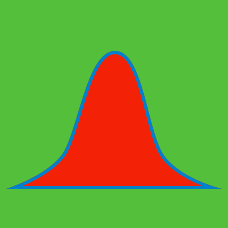Probability

# Exponential Distribution

The probability density function for an Exponential distribution with parameter $\lambda > 0$ is given by

$f(x) = \begin{cases} \lambda e^{-\lambda x} & x \ge 0, \\ 0 & x < 0 \end{cases}.$

At what value of $x$ is the probability density function maximized?

What is the expected value of a random variable with probability density function

$f(x) = \begin{cases} 2e^{-2x} & x \ge 0 \\ 0 & x < 0 \end{cases}?$

Let $X$ be an Exponentially distributed random variable with parameter $\lambda = 3.$ What is the probability that $X=3$?

Suppose that you model the time between when you get email as an Exponentially distributed random variable with mean 10 minutes. You get an email at 2:10pm, then check your email at 2:20pm. Unfortunately, you haven't received another email since. Knowing this, what is the expected value of the number of minutes after 2:20pm at which the next email will arrive?

We continue to assume that the time between emails arriving in your inbox is distributed Exponentially with $\lambda = 1/10$ minutes. Suppose that it's currently 3:00p and an email arrives. You commit to staring at your inbox until two more emails have arrived. What is the expected value of the amount of time you'll be waiting in minutes?

×